## 8.1 Pennies activity

As we did in Chapter 7, we’ll begin with a hands-on tactile activity.

### 8.1.1 What is the average year on US pennies in 2019?

Try to imagine all the pennies being used in the United States in 2019. That’s a lot of pennies! Now say we’re interested in the average year of minting of all these pennies. One way to compute this value would be to gather up all pennies being used in the US, record the year, and compute the average. However, this would be near impossible! So instead, let’s collect a sample of 50 pennies from a local bank in downtown Northampton, Massachusetts, USA as seen in Figure 8.1.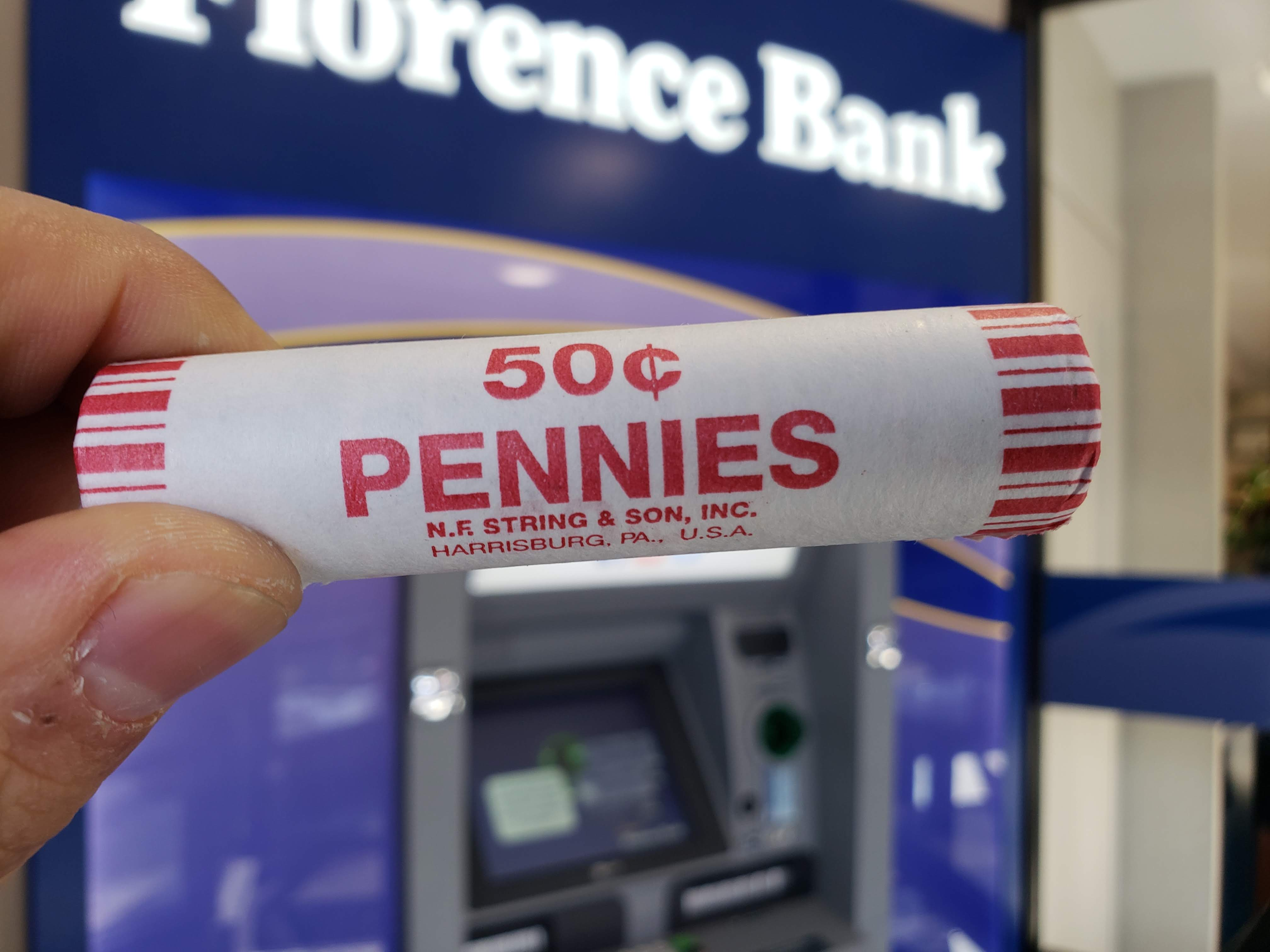FIGURE 8.1: Collecting a sample of 50 US pennies from a local bank.

An image of these 50 pennies can be seen in Figure 8.2. For each of the 50 pennies starting in the top left, progressing row-by-row, and ending in the bottom right, we assigned an “ID” identification variable and marked the year of minting.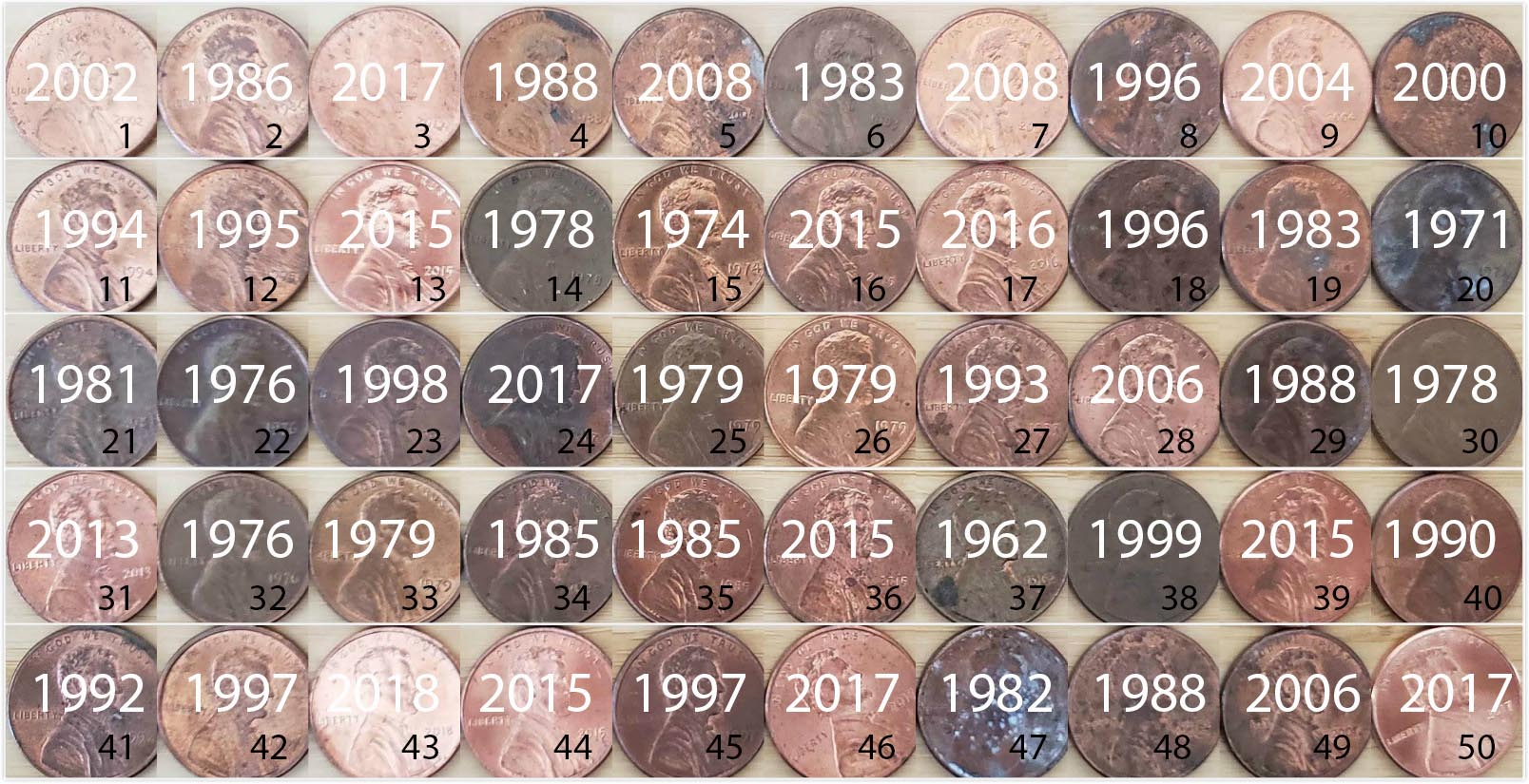FIGURE 8.2: 50 US pennies labelled.

The moderndive package contains this data on our 50 sampled pennies in the pennies_sample data frame:

pennies_sample
# A tibble: 50 x 2
ID  year
<int> <dbl>
1     1  2002
2     2  1986
3     3  2017
4     4  1988
5     5  2008
6     6  1983
7     7  2008
8     8  1996
9     9  2004
10    10  2000
# … with 40 more rows

The pennies_sample data frame has 50 rows corresponding to each penny with two variables. The first variable ID corresponds to the ID labels in Figure 8.2, whereas the second variable year corresponds to the year of minting saved as a numeric variable, also known as a double (dbl).

Based on these 50 sampled pennies, what can we say about all US pennies in 2019? Let’s study some properties of our sample by performing an exploratory data analysis. Let’s first visualize the distribution of the year of these 50 pennies using our data visualization tools from Chapter 2. Since year is a numerical variable, we use a histogram in Figure 8.3 to visualize its distribution.

ggplot(pennies_sample, aes(x = year)) +
geom_histogram(binwidth = 10, color = "white")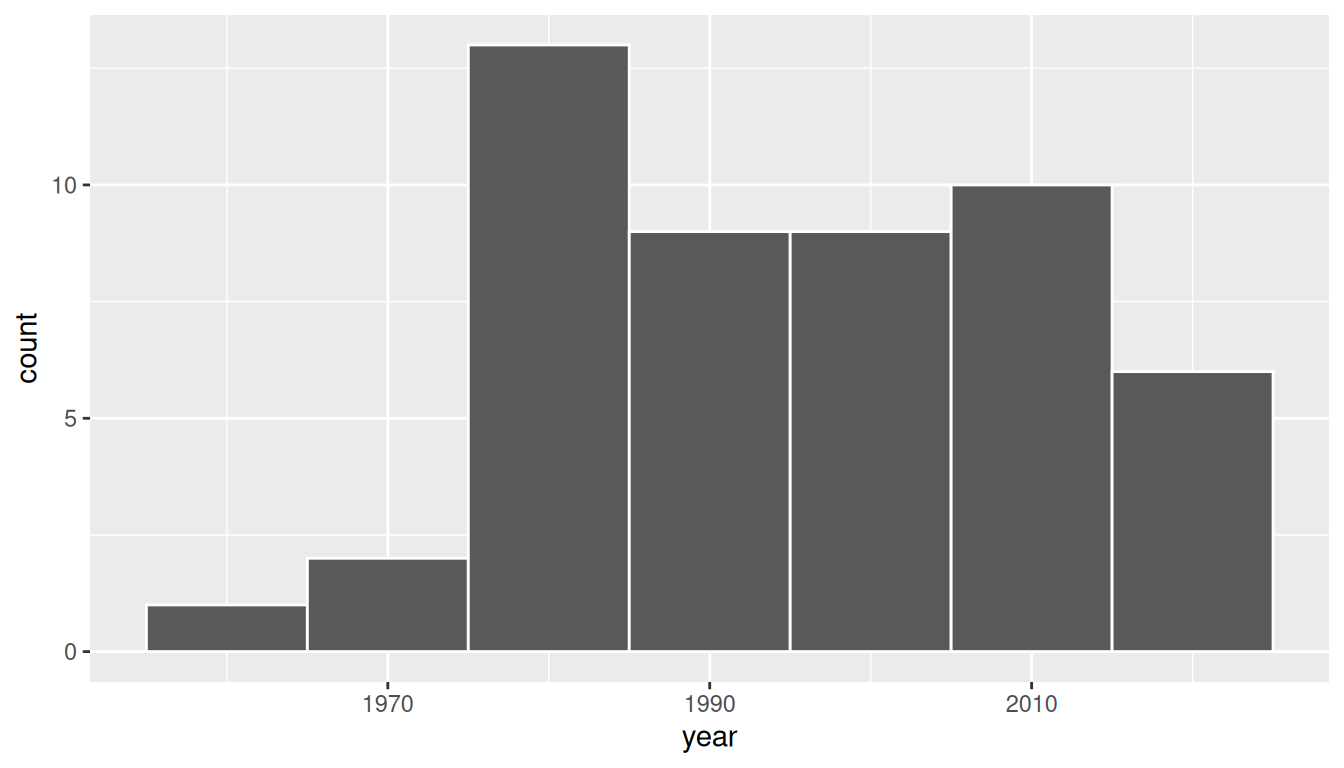FIGURE 8.3: Distribution of year on 50 US pennies.

Observe a slightly left-skewed distribution, since most pennies fall between 1980 and 2010 with only a few pennies older than 1970. What is the average year for the 50 sampled pennies? Eyeballing the histogram it appears to be around 1990. Let’s now compute this value exactly using our data wrangling tools from Chapter 3.

x_bar <- pennies_sample %>%
summarize(mean_year = mean(year))
x_bar
# A tibble: 1 x 1
mean_year
<dbl>
1   1995.44

Thus, if we’re willing to assume that pennies_sample is a representative sample from all US pennies, a “good guess” of the average year of minting of all US pennies would be 1995.44. In other words, around 1995. This should all start sounding similar to what we did previously in Chapter 7!

In Chapter 7, our study population was the bowl of $$N$$ = 2400 balls. Our population parameter was the population proportion of these balls that were red, denoted by $$p$$. In order to estimate $$p$$, we extracted a sample of 50 balls using the shovel. We then computed the relevant point estimate: the sample proportion of these 50 balls that were red, denoted mathematically by $$\widehat{p}$$.

Here our population is $$N$$ = whatever the number of pennies are being used in the US, a value which we don’t know and probably never will. The population parameter of interest is now the population mean year of all these pennies, a value denoted mathematically by the Greek letter $$\mu$$ (pronounced “mu”). In order to estimate $$\mu$$, we went to the bank and obtained a sample of 50 pennies and computed the relevant point estimate: the sample mean year of these 50 pennies, denoted mathematically by $$\overline{x}$$ (pronounced “x-bar”). An alternative and more intuitive notation for the sample mean is $$\widehat{\mu}$$. However, this is unfortunately not as commonly used, so in this book we’ll stick with convention and always denote the sample mean as $$\overline{x}$$.

We summarize the correspondence between the sampling bowl exercise in Chapter 7 and our pennies exercise in Table 8.1, which are the first two rows of the previously seen Table 7.5.

TABLE 8.1: Scenarios of sampling for inference
Scenario Population parameter Notation Point estimate Symbol(s)
1 Population proportion $$p$$ Sample proportion $$\widehat{p}$$
2 Population mean $$\mu$$ Sample mean $$\overline{x}$$ or $$\widehat{\mu}$$

Going back to our 50 sampled pennies in Figure 8.2, the point estimate of interest is the sample mean $$\overline{x}$$ of 1995.44. This quantity is an estimate of the population mean year of all US pennies $$\mu$$.

Recall that we also saw in Chapter 7 that such estimates are prone to sampling variation. For example, in this particular sample in Figure 8.2, we observed three pennies with the year 1999. If we sampled another 50 pennies, would we observe exactly three pennies with the year 1999 again? More than likely not. We might observe none, one, two, or maybe even all 50! The same can be said for the other 26 unique years that are represented in our sample of 50 pennies.

To study the effects of sampling variation in Chapter 7, we took many samples, something we could easily do with our shovel. In our case with pennies, however, how would we obtain another sample? By going to the bank and getting another roll of 50 pennies.

Say we’re feeling lazy, however, and don’t want to go back to the bank. How can we study the effects of sampling variation using our single sample? We will do so using a technique known as bootstrap resampling with replacement, which we now illustrate.

### 8.1.2 Resampling once

Step 1: Let’s print out identically sized slips of paper representing our 50 pennies as seen in Figure 8.4.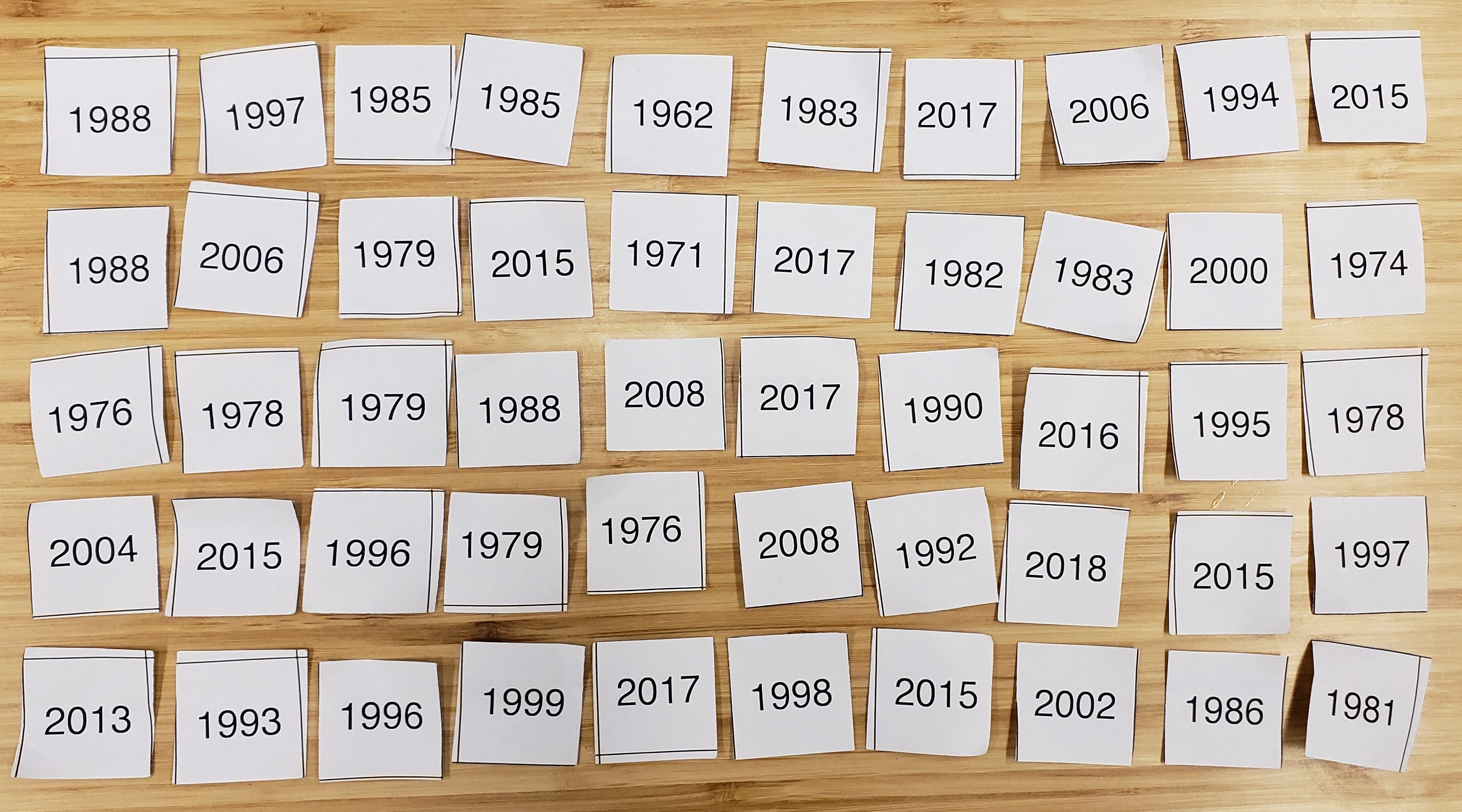FIGURE 8.4: Step 1: 50 slips of paper representing 50 US pennies.

Step 2: Put the 50 slips of paper into a hat or tuque as seen in Figure 8.5.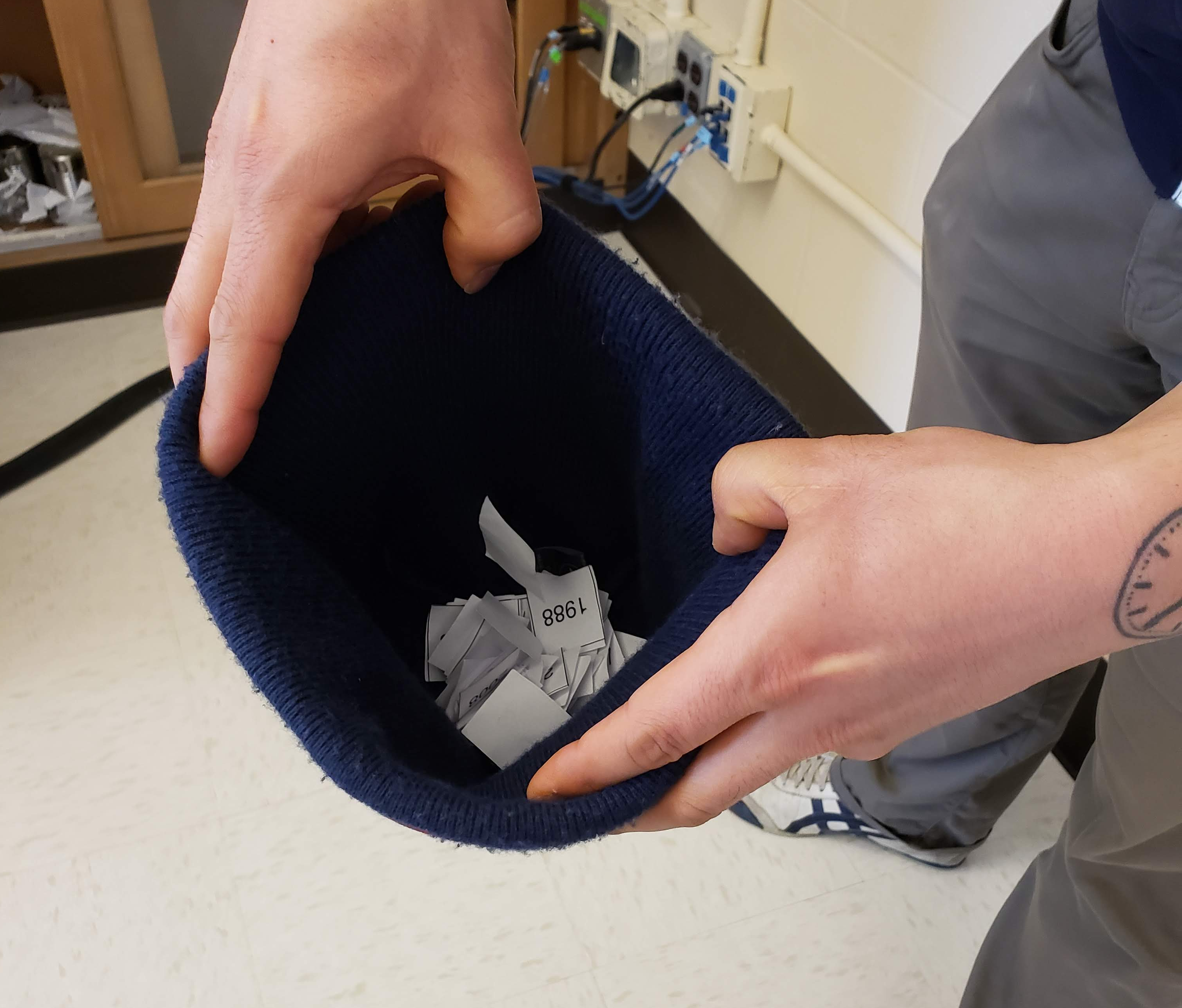FIGURE 8.5: Step 2: Putting 50 slips of paper in a hat.

Step 3: Mix the hat’s contents and draw one slip of paper at random as seen in Figure 8.6. Record the year.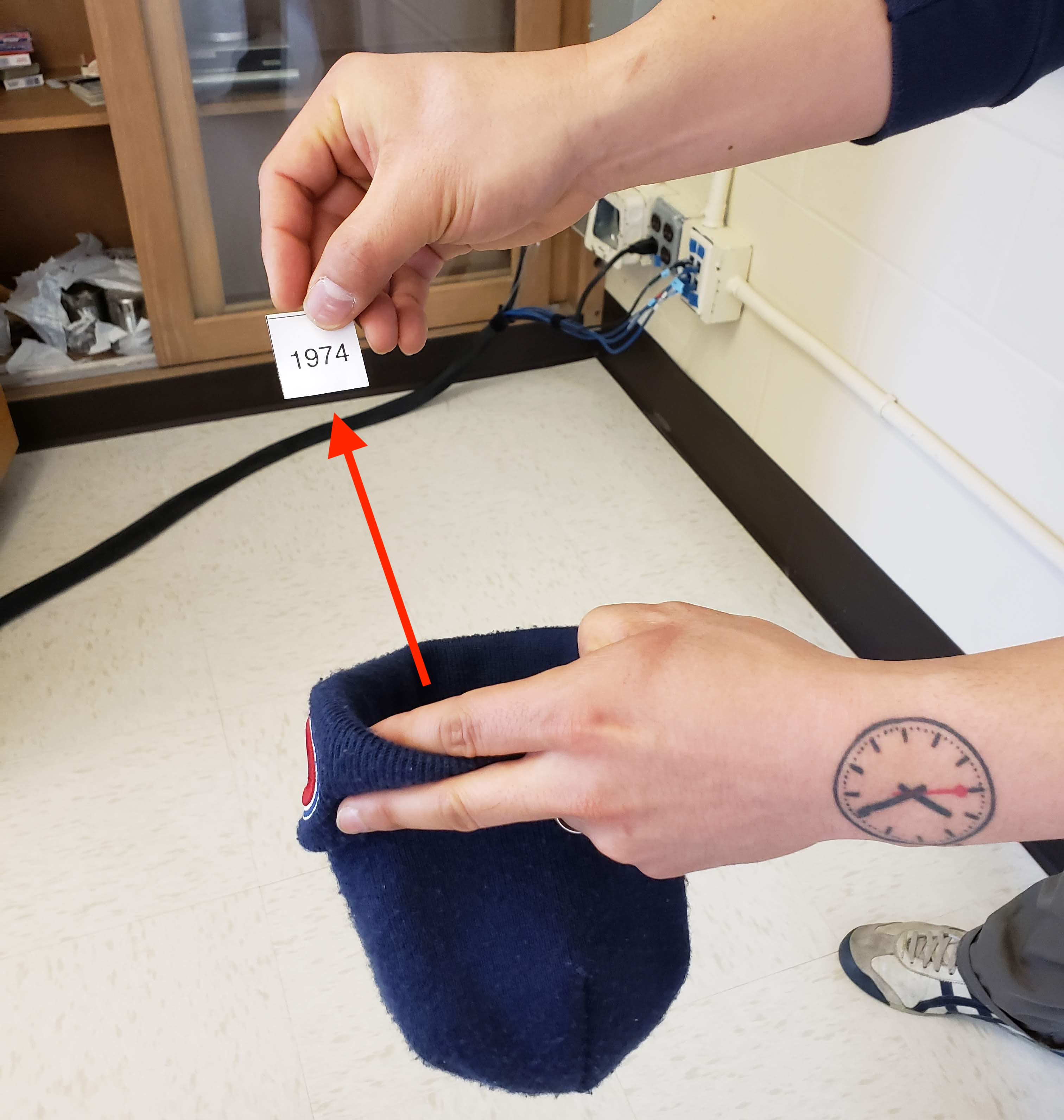FIGURE 8.6: Step 3: Drawing one slip of paper at random.

Step 4: Put the slip of paper back in the hat! In other words, replace it as seen in Figure 8.7.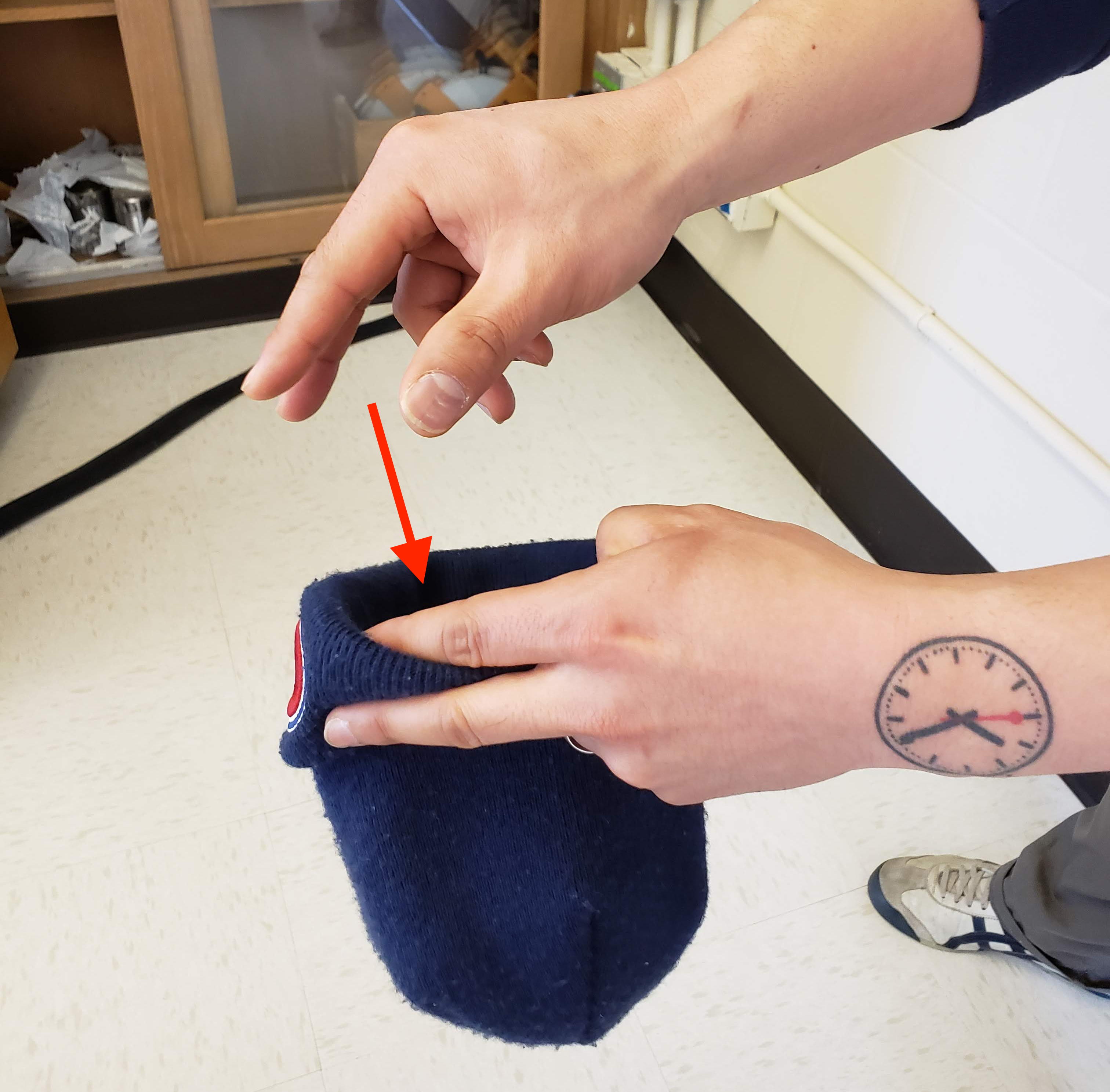FIGURE 8.7: Step 4: Replacing slip of paper.

Step 5: Repeat Steps 3 and 4 a total of 49 more times, resulting in 50 recorded years.

What we just performed was a resampling of the original sample of 50 pennies. We are not sampling 50 pennies from the population of all US pennies as we did in our trip to the bank. Instead, we are mimicking this act by resampling 50 pennies from our original sample of 50 pennies.

Now ask yourselves, why did we replace our resampled slip of paper back into the hat in Step 4? Because if we left the slip of paper out of the hat each time we performed Step 4, we would end up with the same 50 original pennies! In other words, replacing the slips of paper induces sampling variation.

Being more precise with our terminology, we just performed a resampling with replacement from the original sample of 50 pennies. Had we left the slip of paper out of the hat each time we performed Step 4, this would be resampling without replacement.

Let’s study our 50 resampled pennies via an exploratory data analysis. First, let’s load the data into R by manually creating a data frame pennies_resample of our 50 resampled values. We’ll do this using the tibble() command from the dplyr package. Note that the 50 values you resample will almost certainly not be the same as ours given the inherent randomness.

pennies_resample <- tibble(
year = c(1976, 1962, 1976, 1983, 2017, 2015, 2015, 1962, 2016, 1976,
2006, 1997, 1988, 2015, 2015, 1988, 2016, 1978, 1979, 1997,
1974, 2013, 1978, 2015, 2008, 1982, 1986, 1979, 1981, 2004,
2000, 1995, 1999, 2006, 1979, 2015, 1979, 1998, 1981, 2015,
2000, 1999, 1988, 2017, 1992, 1997, 1990, 1988, 2006, 2000)
)

The 50 values of year in pennies_resample represent a resample of size 50 from the original sample of 50 pennies. We display the 50 resampled pennies in Figure 8.8.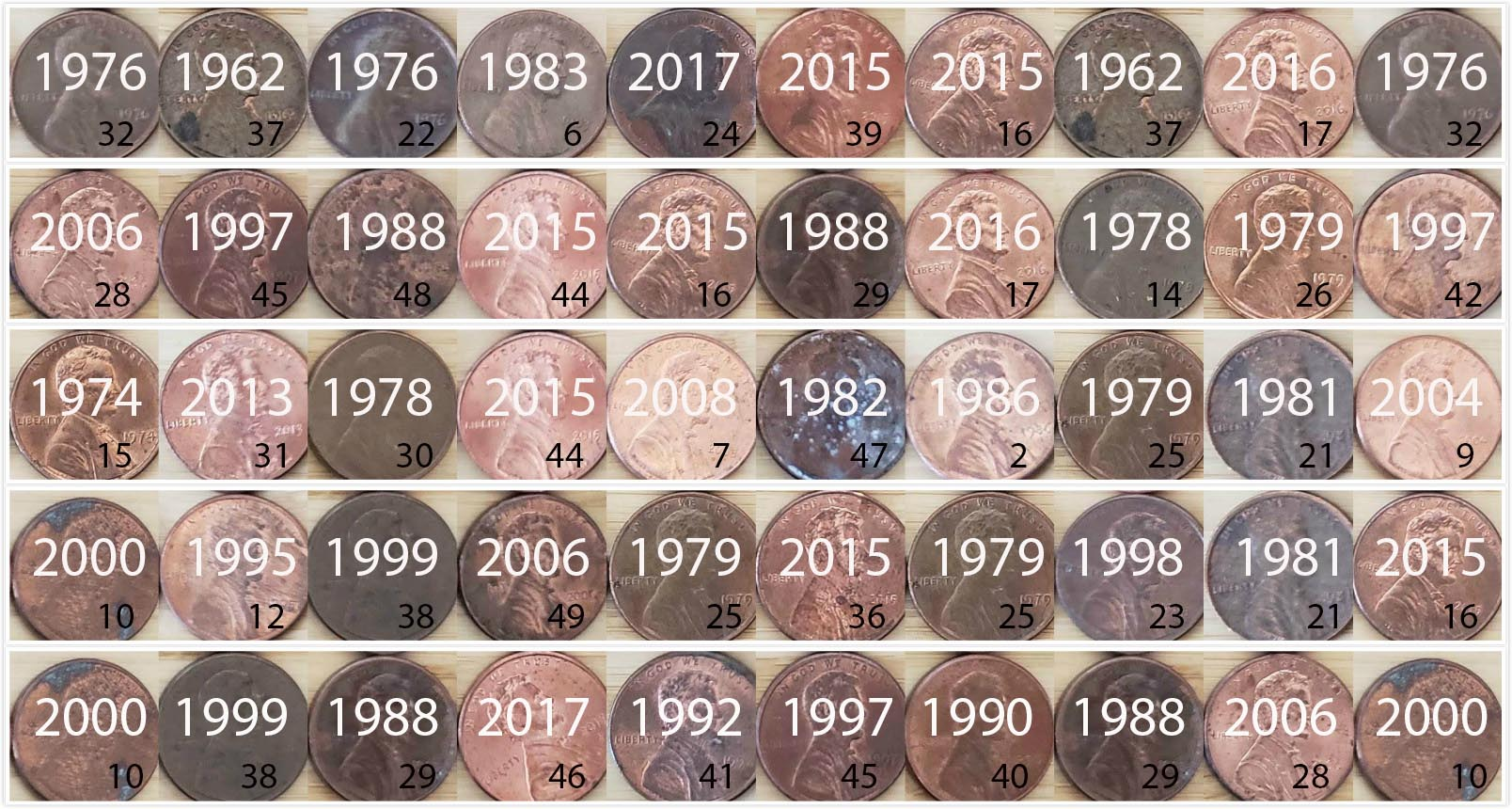FIGURE 8.8: 50 resampled US pennies labelled.

Let’s compare the distribution of the numerical variable year of our 50 resampled pennies with the distribution of the numerical variable year of our original sample of 50 pennies in Figure 8.9.

ggplot(pennies_resample, aes(x = year)) +
geom_histogram(binwidth = 10, color = "white") +
labs(title = "Resample of 50 pennies")
ggplot(pennies_sample, aes(x = year)) +
geom_histogram(binwidth = 10, color = "white") +
labs(title = "Original sample of 50 pennies")FIGURE 8.9: Comparing year in the resampled pennies_resample with the original sample pennies_sample.

Observe in Figure 8.9 that while the general shapes of both distributions of year are roughly similar, they are not identical.

Recall from the previous section that the sample mean of the original sample of 50 pennies from the bank was 1995.44. What about for our resample? Any guesses? Let’s have dplyr help us out as before:

pennies_resample %>%
summarize(mean_year = mean(year))
# A tibble: 1 x 1
mean_year
<dbl>
1      1996

We obtained a different mean year of 1996. This variation is induced by the resampling with replacement we performed earlier.

What if we repeated this resampling exercise many times? Would we obtain the same mean year each time? In other words, would our guess at the mean year of all pennies in the US in 2019 be exactly 1996 every time? Just as we did in Chapter 7, let’s perform this resampling activity with the help of some of our friends: 35 friends in total.

### 8.1.3 Resampling 35 times

Each of our 35 friends will repeat the same five steps:

1. Start with 50 identically sized slips of paper representing the 50 pennies.
2. Put the 50 small pieces of paper into a hat or beanie cap.
3. Mix the hat’s contents and draw one slip of paper at random. Record the year in a spreadsheet.
4. Replace the slip of paper back in the hat!
5. Repeat Steps 3 and 4 a total of 49 more times, resulting in 50 recorded years.

Since we had 35 of our friends perform this task, we ended up with $$35 \cdot 50 = 1750$$ values. We recorded these values in a shared spreadsheet with 50 rows (plus a header row) and 35 columns. We display a snapshot of the first 10 rows and five columns of this shared spreadsheet in Figure 8.10.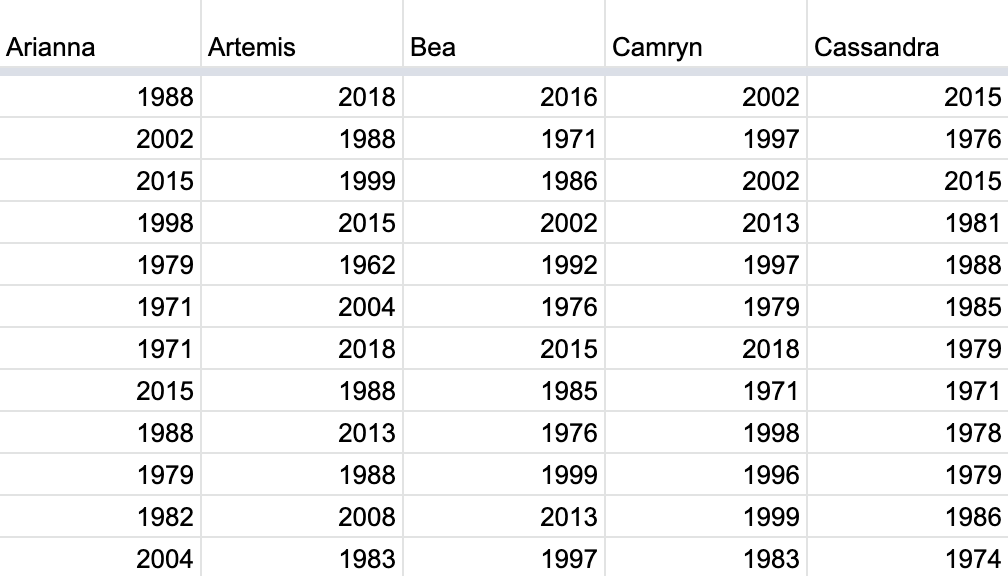FIGURE 8.10: Snapshot of shared spreadsheet of resampled pennies.

For your convenience, we’ve taken these 35 $$\cdot$$ 50 = 1750 values and saved them in pennies_resamples, a “tidy” data frame included in the moderndive package. We saw what it means for a data frame to be “tidy” in Subsection 4.2.1.

pennies_resamples
# A tibble: 1,750 x 3
# Groups:   name 
replicate name     year
<int> <chr>   <dbl>
1         1 Arianna  1988
2         1 Arianna  2002
3         1 Arianna  2015
4         1 Arianna  1998
5         1 Arianna  1979
6         1 Arianna  1971
7         1 Arianna  1971
8         1 Arianna  2015
9         1 Arianna  1988
10         1 Arianna  1979
# … with 1,740 more rows

What did each of our 35 friends obtain as the mean year? Once again, dplyr to the rescue! After grouping the rows by name, we summarize each group of 50 rows by their mean year:

resampled_means <- pennies_resamples %>%
group_by(name) %>%
summarize(mean_year = mean(year))
resampled_means
# A tibble: 35 x 2
name      mean_year
<chr>         <dbl>
1 Arianna     1992.5
2 Artemis     1996.42
3 Bea         1996.32
4 Camryn      1996.9
5 Cassandra   1991.22
6 Cindy       1995.48
7 Claire      1995.52
8 Dahlia      1998.48
9 Dan         1993.86
10 Eindra      1993.56
# … with 25 more rows

Observe that resampled_means has 35 rows corresponding to the 35 means based on the 35 resamples. Furthermore, observe the variation in the 35 values in the variable mean_year. Let’s visualize this variation using a histogram in Figure 8.11. Recall that adding the argument boundary = 1990 to the geom_histogram() sets the binning structure so that one of the bin boundaries is at 1990 exactly.

ggplot(resampled_means, aes(x = mean_year)) +
geom_histogram(binwidth = 1, color = "white", boundary = 1990) +
labs(x = "Sampled mean year")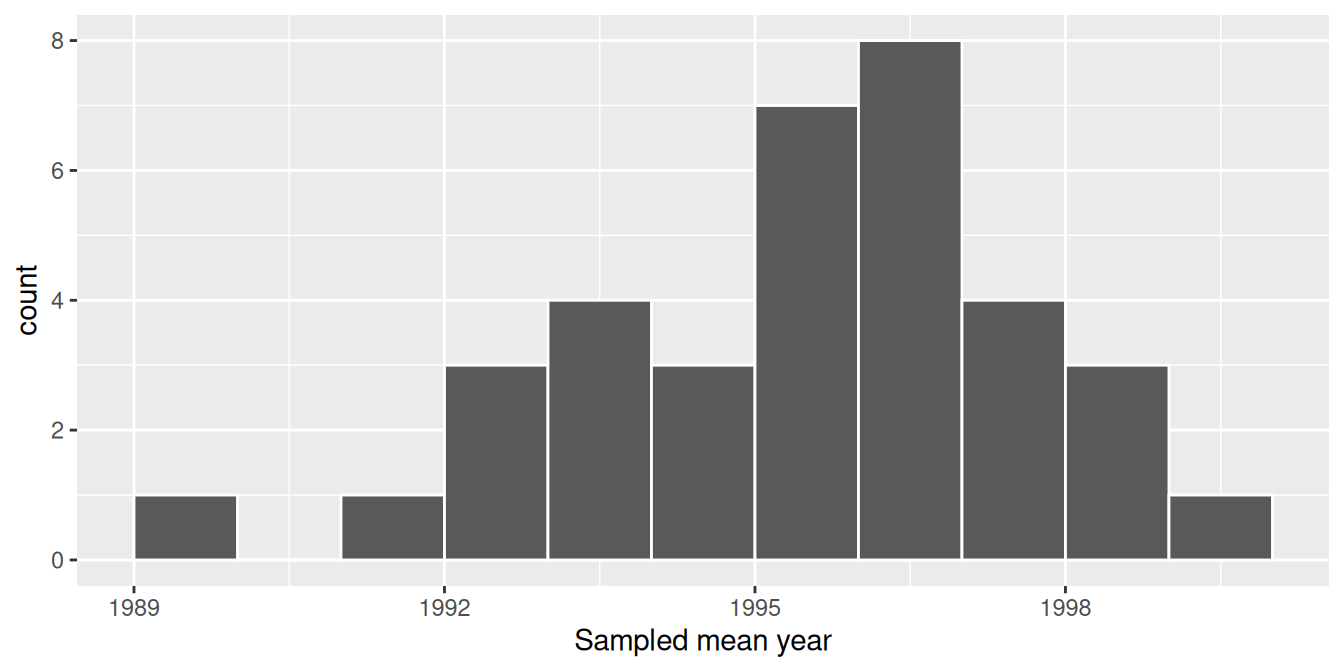FIGURE 8.11: Distribution of 35 sample means from 35 resamples.

Observe in Figure 8.11 that the distribution looks roughly normal and that we rarely observe sample mean years less than 1992 or greater than 2000. Also observe how the distribution is roughly centered at 1995, which is close to the sample mean of 1995.44 of the original sample of 50 pennies from the bank.

### 8.1.4 What did we just do?

What we just demonstrated in this activity is the statistical procedure known as bootstrap resampling with replacement. We used resampling to mimic the sampling variation we studied in Chapter 7 on sampling. However, in this case, we did so using only a single sample from the population.

In fact, the histogram of sample means from 35 resamples in Figure 8.11 is called the bootstrap distribution. It is an approximation to the sampling distribution of the sample mean, in the sense that both distributions will have a similar shape and similar spread. In fact in the upcoming Section 8.7, we’ll show you that this is the case. Using this bootstrap distribution, we can study the effect of sampling variation on our estimates. In particular, we’ll study the typical “error” of our estimates, known as the standard error.

In Section 8.2 we’ll mimic our tactile resampling activity virtually on the computer, allowing us to quickly perform the resampling many more than 35 times. In Section 8.3 we’ll define the statistical concept of a confidence interval, which builds off the concept of bootstrap distributions.

In Section 8.4, we’ll construct confidence intervals using the dplyr package, as well as a new package: the infer package for “tidy” and transparent statistical inference. We’ll introduce the “tidy” statistical inference framework that was the motivation for the infer package pipeline. The infer package will be the driving package throughout the rest of this book.

As we did in Chapter 7, we’ll tie all these ideas together with a real-life case study in Section 8.6. This time we’ll look at data from an experiment about yawning from the US television show Mythbusters.